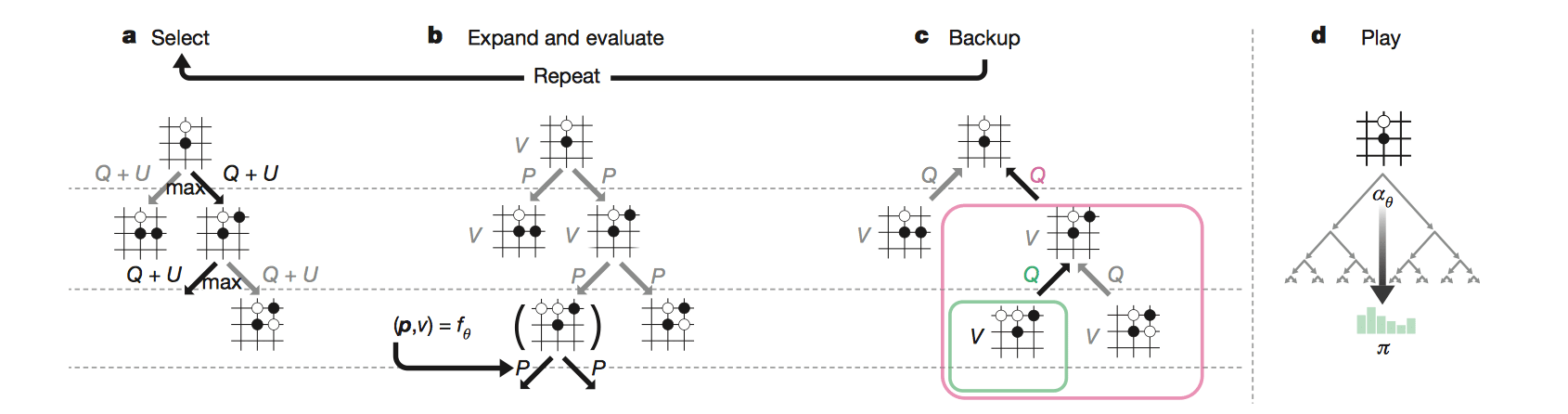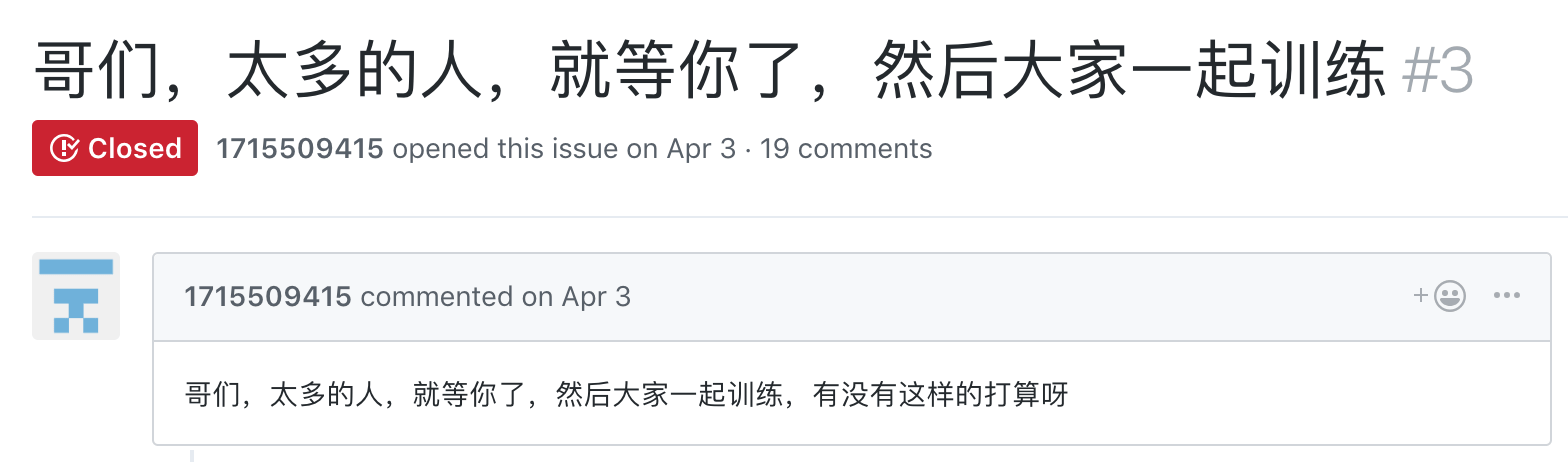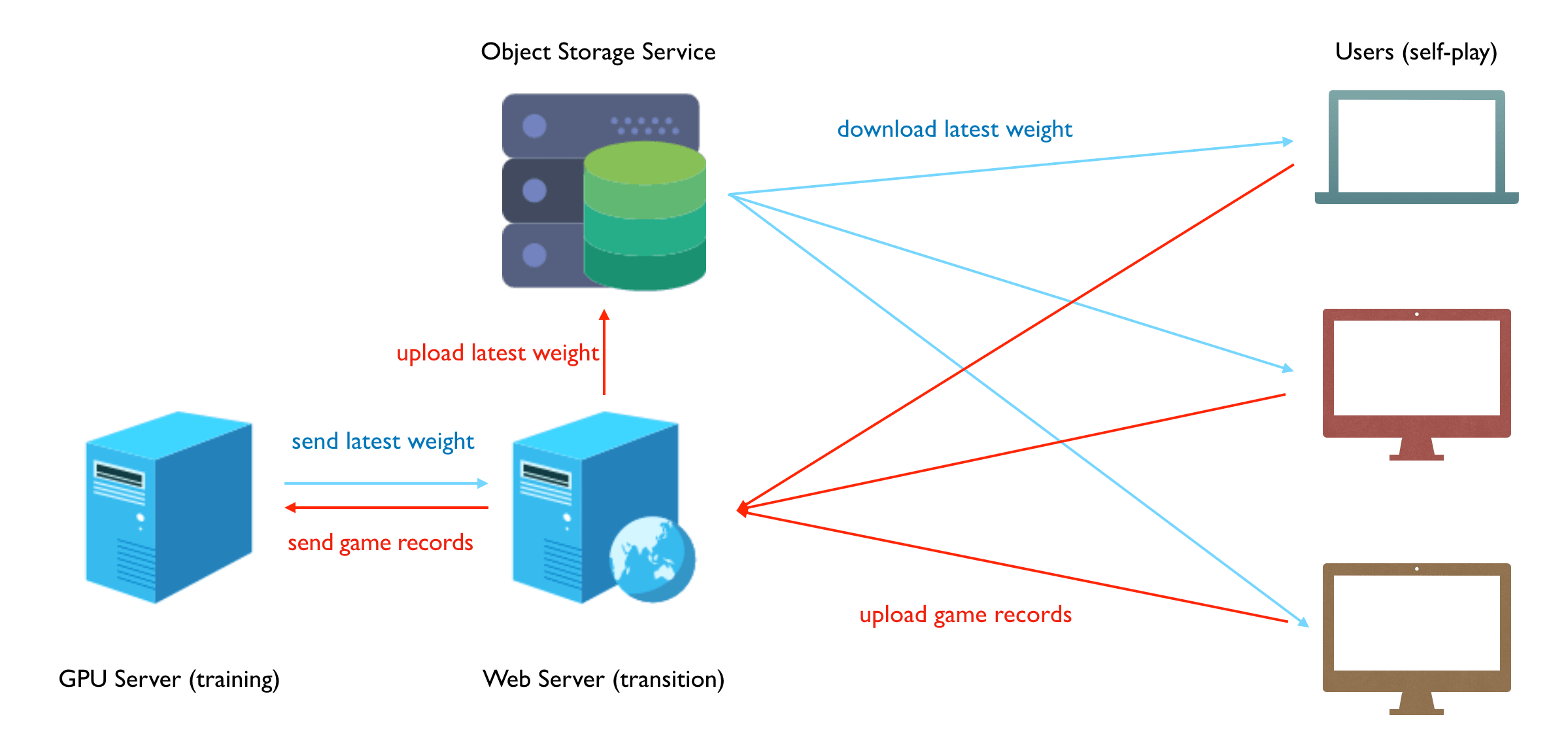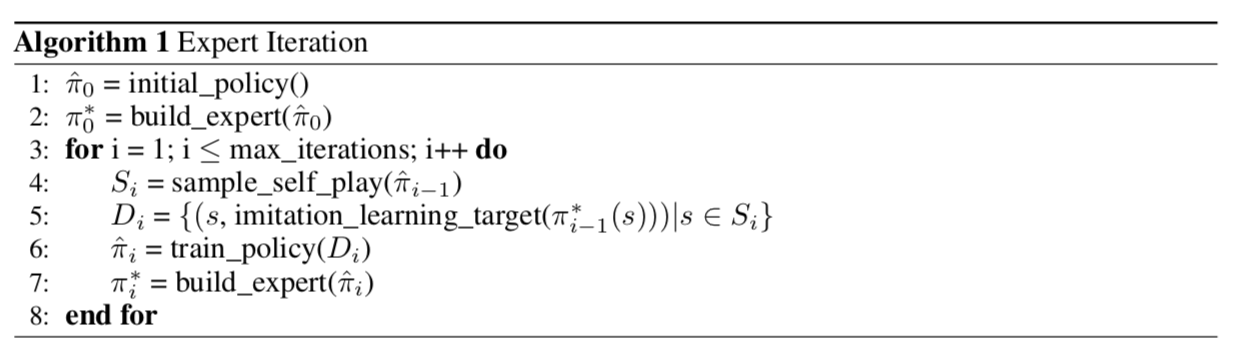# NIUHE

## 模块

• agents：核心模块，决定如何下棋
• model.py：神经网络模型
• player.py：MCTS，输出走法
• api.py：供外界调用model
• envrionment：象棋规则
• 训练（跑谱）使用static_env.py，速度快一些
• 用自带GUI下棋时使用的是env.py, chessboard.py这些，可以输出PNG格式的棋谱
• worker：把agent和envrionment串联起来的脚本
• self_play.py：自我博弈
• compute_elo.py：评测并上传结果到官网
• optimize.py：训练棋谱
• _windows后缀表示是在Windows平台上运行的相应功能，之所以分开是因为两个多进程的启动方式不同，导致代码结构也要发生一些变化，详见自我博弈

### 神经网络模型

• $10 \times 9$ 是中国象棋棋盘的大小
• $14$ 是所有棋子种类（红/黑算不同种类）
• 整体的输入就是14个棋盘堆叠在一起，每个棋盘表示一种棋子的位置：棋子所在的位置为1，其余位置为0。

• 策略头（policy head）输出：$2086$
• $2086$ 是行动空间的大小。行动空间就是说根据中国象棋的规则，任意棋子在任意位置的走法集合。
• 价值头（value head）输出：$1$
• 价值头输出一个标量衡量当前局势 $v\in[-1, 1]$：当 $v$ 接近1时，局势大好；接近0为均势；接近-1为败势。

1
2
3

### 蒙特卡洛树搜索1. 选择

每个模拟的第一个 in-tree 阶段开始于搜索树的根节点 $s_0$，并且在模拟时刻 L 处到达叶节点 $s_L$ 时结束。在每个这些时刻 $t < L$ 处，根据搜索树中的统计量选择一个移动: $a_t = \arg\max_a(Q(s_t,a) + U(s_t,a))$，其中 $U(s_t,a)$ 使用PUCT算法的变体得到 $U(s,a)=c_{puct}P(s,a)\frac{\sqrt{\sum_bN(s,b)}}{1+N(s,a)}$ 其中 $c_{puct}​$ 是一个决定探索程度的常数; 这种搜索控制策略最初倾向于具有高先验概率和低访问次数的行为，但后期倾向于具有高动作价值的行为。

2. 扩展和评估

叶子结点 $s_L$ 被加入到等待评估队列进行评估: $(d_i(p),v)=f_\theta(d_i(s_L))$，其中 $d_i$是旋转或反射操作。神经网络一次评估队列里的 8 个结点;搜索进程直到评估完毕才能继续工作。每个叶子结点和每条边 $(s_L,a)$ 的统计值被初始化为 $\{N(s_L,a) = 0,W(s_L,a) = 0,Q(s_L,a) =0, P(s_L, a) = p_a\}$，然后价值 v 开始回溯。

3. 回溯

每条边的统计值延路径反向更新：访问计数递增 $N(s_t,𝑎_t) = N(s_t,𝑎_t) +1$，移动价值更新为平均值 $W(s_t,a_t)=W(s_t,a_t)+v$, $Q(s_t,a_t)=\frac{W(s_t,a_t)}{N(s_t,a_t)}$

4. 下棋

在搜索结束时，AlphaGo Zero 在根位置 $s_0$ 选择移动 a，与其指数访问计数成比例，$\pi(a|s_0) = \frac{N(s_0,a)^{1/\tau}}{\sum_bN(s,b)^{1/\tau}}$，其中 $τ$ 是控制探索水平的参数。搜索树可以在后面的时刻重用：与所选择的移动对应的子节点成为新的根节点; 在这个节点下面的子树被保留以及它的所有统计数据，而树的其余部分被丢弃。

#### 实现细节

• 根据比赛规则：闲着循环3次判和；违规（长捉、长将等）判负；对方违规判胜。

Virtual Loss

• 多线程搜索时，当某一线程选择了某个action时，为了鼓励其他线程选择其他action，应该降低该action的价值（施加virtual loss）

• 在回溯时，更新value要考虑到virtual loss的影响

state表示

Forsyth–Edwards Notation (FEN) is a standard notation for describing a particular board position of a chess game. The purpose of FEN is to provide all the necessary information to restart a game from a particular position.

### 自我博弈

On Windows only 'spawn' is available. On Unix 'fork' and 'spawn' are always supported, with 'fork' being the default.

## 分布式1. 首先是帮忙跑谱的大多都是象棋爱好者，并非开发者，所以我要把Python代码打包成exe文件分发给他们一键执行，最终使用PyInstaller打包成功，这其中遇到了很多坑：
• 卸载cytoolz；pandas的版本必须为0.20.3
• 代码里加上mp.freeze_support()，否则多进程不会正常工作
2. 服务器带宽有限，客户端下载权重太慢，解决办法：把权重放到云存储服务中，如腾讯云/七牛云的对象存储服务。
3. 中国象棋棋规的完善。并不是说基础的马走日象走田这种规则，而是像长将、长捉等这种比赛规则，这个算是坑最大的一个，直到现在规则还存在少许问题。
4. 部分支持了UCI协议。这样就可以使用其他的象棋界面加载这个引擎，并且能和其他引擎对弈。
5. 因为“同行竞争”，我的服务器在今年暑假期间我的服务器经常遭受DDos攻击，由于买不起腾讯云的高防服务，只能尝试其他办法，包括配置弹性IP、配置防火墙、Cloudfare CDN等，但都不好用。最终把服务转移到OVH提供的VPS上才解决了问题（OVH提供免费的DDos防护）。

## AlphaZero and ExIt

Expert Iteration（ExIt）是一种模仿学习（Imitation Learning, IL）算法，普通的 IL 算法中，徒弟模仿专家的策略只能提高自己的策略，专家是不会有任何提高的，而 ExIt 算法就是想让师傅教徒弟的时候自己也有提高。

ExIt 算法 师傅根据徒弟的策略进行前向搜索（例如MCTS，alpha-beta，贪心搜索等），得出比徒弟更好的策略，然后徒弟再学习师傅的策略，如此循环，随着徒弟的增强，师傅也会越来越强。